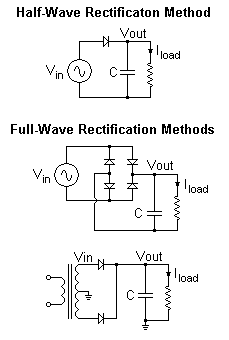# Calculators for Radio Repair and More

This webpage was created with the intention of coalescing several useful formulas from various sources that I commonly use in radio repair or building projects. These calculators do not have much description in their usage besides the quick reference images that are placed at the right if present. The formulas behind each calculator can be easily seen in the Javascript source in the head of the HTML file on this webpage. Hopefully one or more of these calculators below are useful for your purposes.

### Generic Voltage Divider

 Input Voltage Volts Resistor 1 Ohms Resistor 2 Ohms Volts### Resistor Voltage Drop for Load

 DC Supply Voltage Volts Load Voltage Volts Load Current Amperes Ohms Watts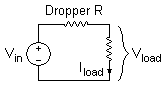### Inductive or Capacitive Reactance

 Component InductorCapacitor Value Henries or Farads Frequency Hertz Reactance Ohms Resonant Component Farads or Henries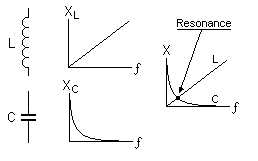### LC Resonant Frequency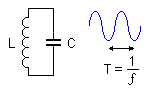### Voltage Drop for Series Tube Filaments

 AC Supply Voltage Volts AC Supply Frequency Hertz Cumulative Filament Voltage Volts Filament Current Amperes Surge Limiter Voltage Drop Volts
 Dropper Capacitor uF Surge Limiter Resistor Ohms Resistor Wattage Watts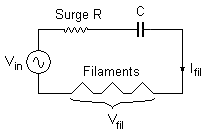Dropper Resistor with diode Ohms Resistor Wattage Watts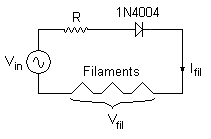Dropper Resistor Ohms Resistor Wattage Watts### Villard Voltage Doubler Output

 AC Supply Voltage Volts AC Supply Frequency Hertz Number of Cascades Output Load Current Amperes Capacitor * Farads Volts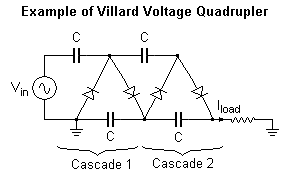* Assumes capacitors in doubler cascades are all the same value.

### Power Supply Output Voltages

 AC Supply Voltage Volts AC Supply Frequency Hertz Output Load Current Amperes Filter Capacitor Farads Rectification Method Half-WaveFull-Wave DC Average Output Voltage Volts AC Ripple Voltage Volts